# Explained: The Concept of Normal Strain

Normal strain, , describes the element level intensity of deformation due to elongation, and it is defined as the increase or decrease in length of an element, due to deformation, divided by the element’s original length.

1. The deformation of some portion of a loaded body may be uniform. Like stress, strain is defined locally. Here again is the sheet on which square elements of equal sizes were drawn. The sheet is elongated by two applied forces. Elements at points B and C have elongated less than and more than point A. But the elongations of all elements around A are equal.

The deformation of some length of the bar around A is said to be uniform. It is easiest to motivate and explain the definition of strain when the deformation is uniform (near A). The definition can be extended to situations (near points B and C) where the deformation is nonuniform.

2. For fixed tensile forces, elongation of a bar increases in proportion to its length. Consider three bars that have identical cross-sections and material properties. Apply identical tensile forces to each. The bars differ only in their lengths: L, 2L, and 3L. Say the deformation is uniform over the whole length of each bar. How do the elongations of the bars differ?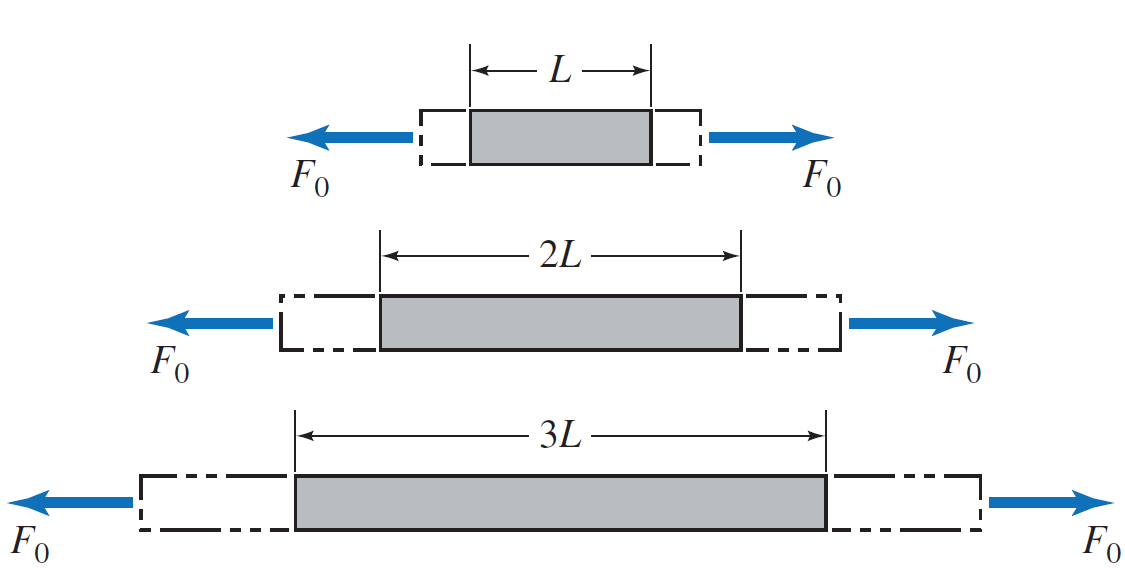Think of each bar as composed of a series of identically sized segments. If the first bar (L) has N segments, there are 2N segments in the second bar, and 3N segments in the third bar. Every segment experiences the same force on the same area and material, and so it elongates the same amount. If the
elongation of the first bar is , the second bar, which has twice as many segments, elongates by 2δ, and the third bar by 3δ. So the elongation is proportional to length.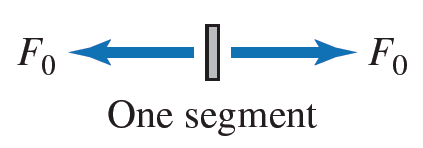3. A segment of a uniformly deforming bar elongates in proportion to the segment’s length. By the same logic, consider a bar of length L that elongates uniformly by . A sub-portion of that bar, having length L1, must elongate by only (L1/L)δ or L1(δ/L). So the ratio δ/L captures the “intensity” or severity of the deformation. From δ/L, the elongation of any portion of a bar may be found. For example, this bar was originally 160 mm long and has elongated by 8 mm.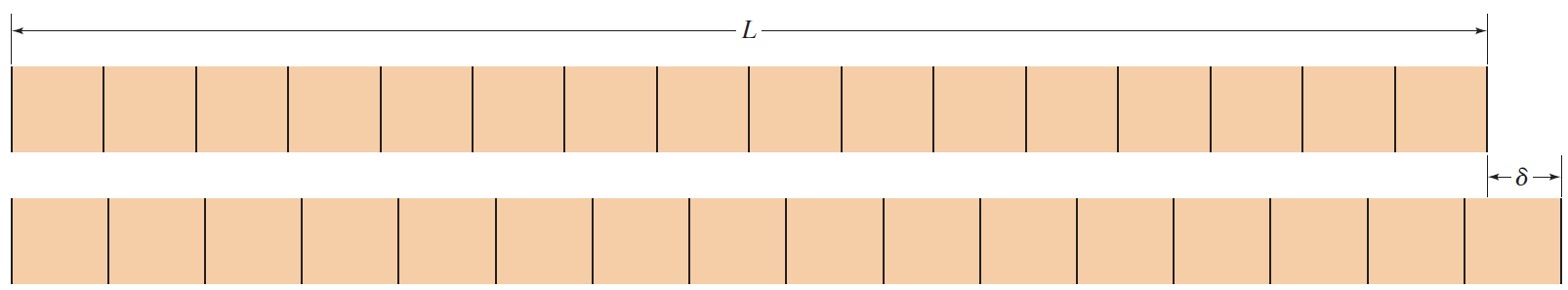Look at one half of the original bar (any segment 8 units in length). Measure its deformed and initial lengths. Their difference, 84 mm - 80 mm, is the elongation, which is 4 mm or one half of the total elongation. Likewise, one quarter of the original bar (any quarter) elongates by 2 mm.
The ratio of δ/L = 8/160= 0.05 describes the deformation in any part of this bar, since 4/80 = 2/40 = 0.05.

4. Normal strain, , is defined as the elongation per initial length, and can refer to an infinitesimal element or to a finite segment of a body. For an infinitesimal element of length ΔL that elongates by Δδ, we define normal strain,ε, by:
ε = Δδ/ΔL
Because the elements in this sheet can be seen to elongate by different amounts, the deformation (strain) in this sheet varies from element to element.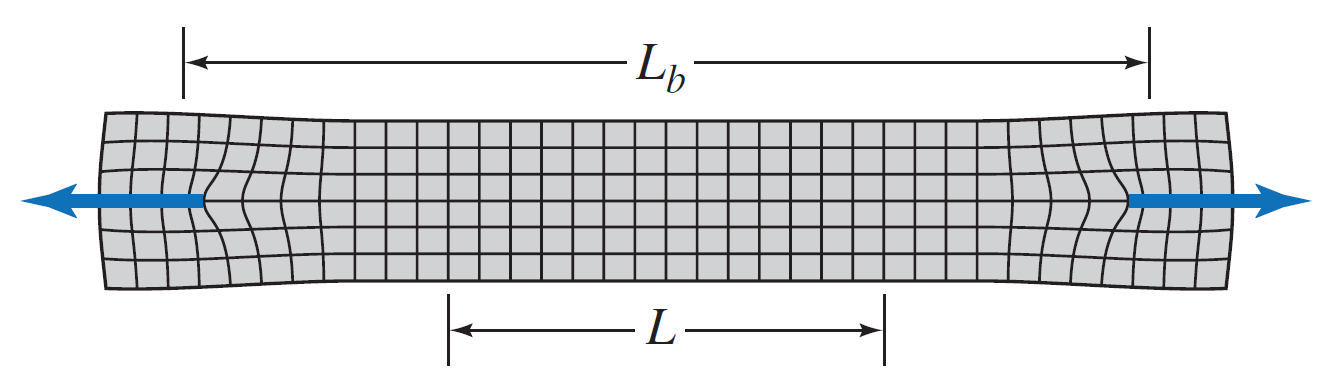The deformation is uniform over the segment of length L. If that segment elongates by δ, then the strain, , at each element in that segment is equal to the segment’s average normal strain:
ε = δ/L
We can still define an average normal strain εavg for the loaded part of the body. If the length Lb elongates by δb, then
ε = δb/Lb
Strain is a pure number with no units, since the units of δ and L are both, say, meters. For machines and structures, strains are usually small ( 10^-5 to 10^-3). To avoid exponents with small numbers, we sometimes use percent strain or micro-strain: 1% strain 0.01 and 1 micro-strain = 10^-6. In some situations, e.g., rubber and some biological tissues, strains can be large (~1 or more).

5. Normal strain describes deformation that is independent of the size of the deformed region and can sometimes be found from measurements. Like with stress, the definition of strain is particularly helpful for two reasons:
• Strain describes the deformation of an element, without the need to specify the size of the element.
• Provided the deformation in some region is uniform, one can compute the strain (equal in all elements in the region) by
ε = δ/L
where L is the length of the elongating region and is its elongation.

6. Strains corresponding to shortening of a body are designated as compressive. Strain is also used to describe element deformation when a body is compressed and shortens. Compressive strain is still change in length (shortening) per initial length. We can specify the strain with a positive number, and state that it is compressive, or we give the strain a negative sign, provided we agree that negative strain means compression.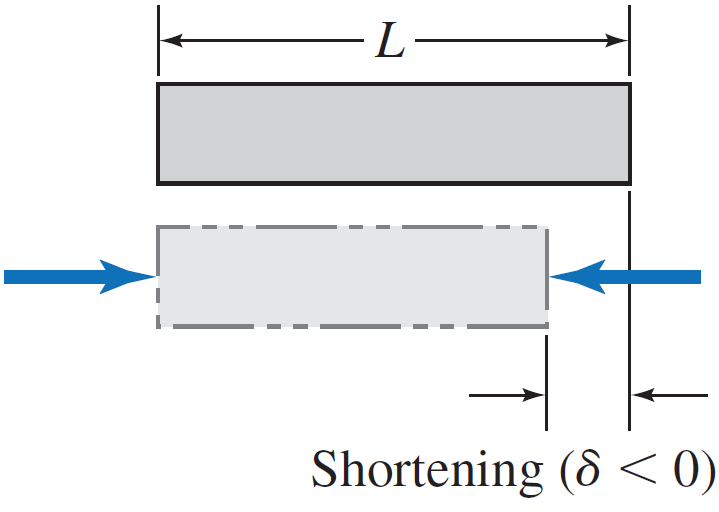7. Don’t confuse strain with elongation and don’t confuse stress with strain.
In engineering, stress quantifies the intensity of force per area that seeks to elongate or shorten an element. Strain quantifies the actual elongation or shortening, normalized by length. As we see next, the same stress can produce very different strains in two bodies of identical dimensions, if they are composed of different materials.